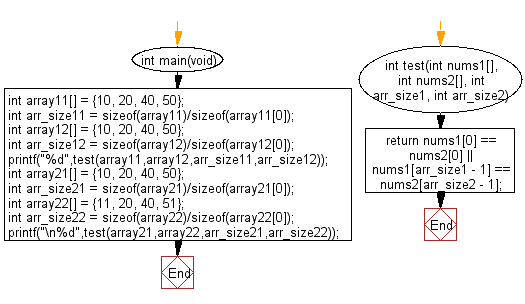﻿ C : Check two arrays have the same first and last element# C Exercises: Check two given arrays of integers of length 1 or more and return true if they have the same first element or they have the same last element

## C-programming basic algorithm: Exercise-35 with Solution

Write a C program to check two given arrays of integers of length 1 or more and return true if they have the same first element or they have the same last element.

C Code:

``````#include <stdio.h>
#include <stdlib.h>

int main(void){

int array11[] = {10, 20, 40, 50};
int arr_size11 = sizeof(array11)/sizeof(array11);
int array12[] = {10, 20, 40, 50};
int arr_size12 = sizeof(array12)/sizeof(array12);
printf("%d",test(array11,array12,arr_size11,arr_size12));

int array21[] = {10, 20, 40, 50};
int arr_size21 = sizeof(array21)/sizeof(array21);
int array22[] = {11, 20, 40, 51};
int arr_size22 = sizeof(array22)/sizeof(array22);
printf("\n%d",test(array21,array22,arr_size21,arr_size22));

}

int test(int nums1[], int nums2[], int arr_size1, int arr_size2)
{
return nums1 == nums2 || nums1[arr_size1 - 1] == nums2[arr_size2 - 1];
}
``````

Sample Output:

```1
0
```

Pictorial Presentation:Flowchart:C Programming Code Editor:

What is the difficulty level of this exercise?

Test your Programming skills with w3resource's quiz.

﻿

## C Programming: Tips of the Day

Why doesn't a+++++b work?

printf("%d",a+++++b); is interpreted as (a++)++ + b according to the Maximal Munch Rule!.

++ (postfix) doesn't evaluate to an lvalue but it requires its operand to be an lvalue.

! 6.4/4 says the next preprocessing token is the longest sequence of characters that could constitute a preprocessing token"

Ref : https://bit.ly/3fdldUT